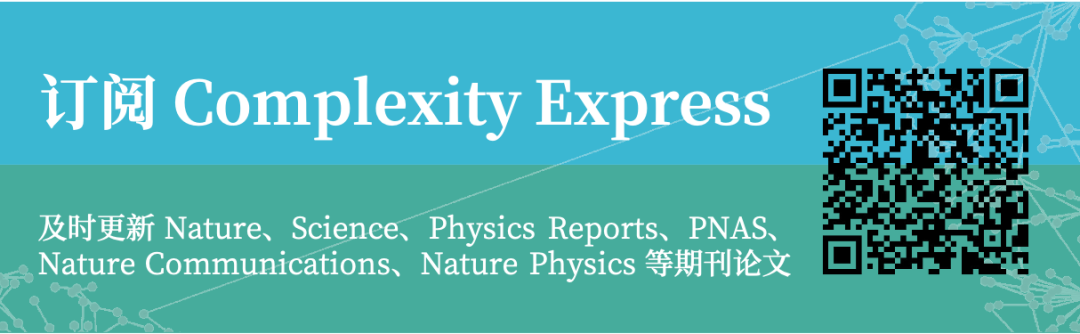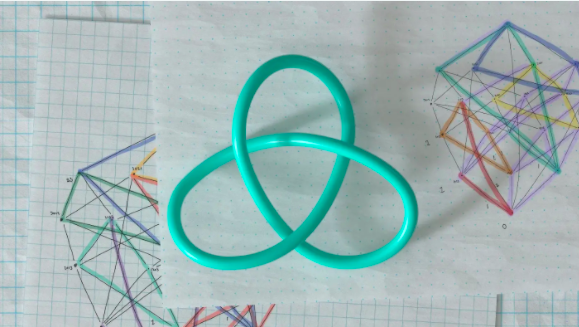Alex Davies, Petar Veličković, Lars Buesing等 | 作者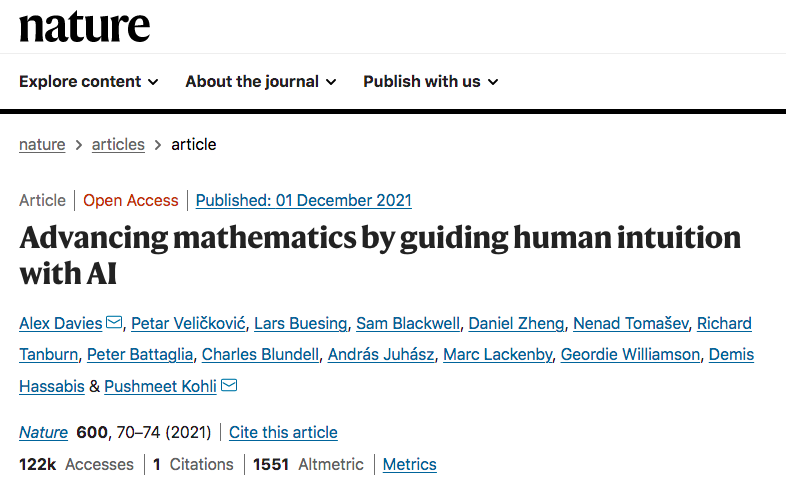Advancing mathematics by guiding human intuition with AI

https://www.nature.com/articles/s41586-021-04086-x

### 3. 使用AI引导数学直觉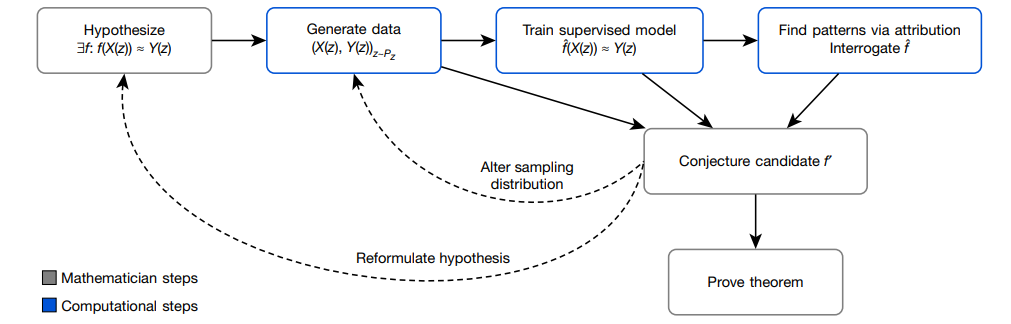*注：例如，我们常见的欧拉公式形式为F-E+V=2，即 -V+E+2=F，V、E、F分别表示顶点、边、面的数量。

### 4. 拓扑学： 纽结理论中代数与几何不变量的关系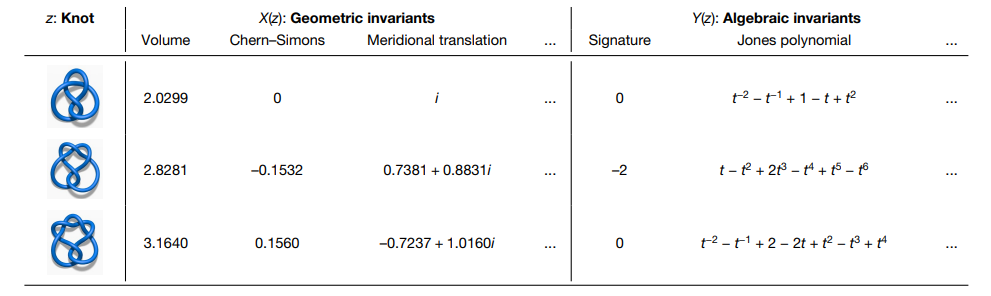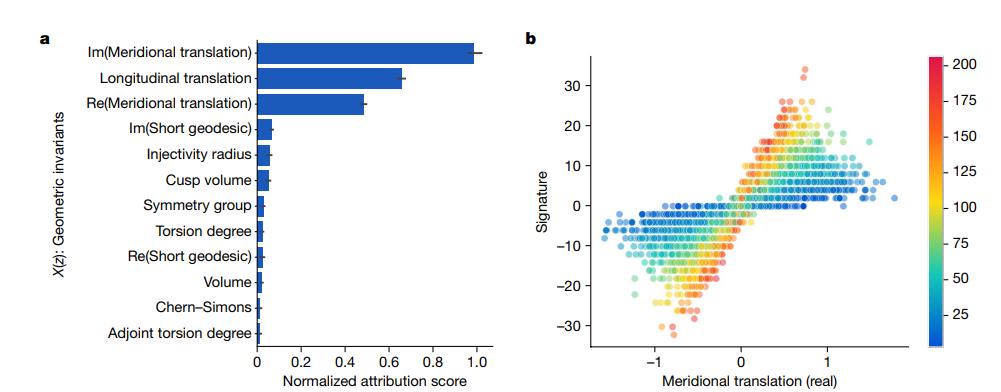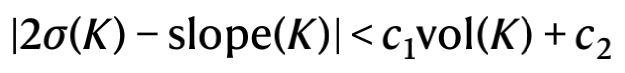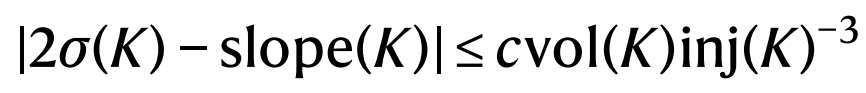### 5. 表示论：对称群组合不变性猜想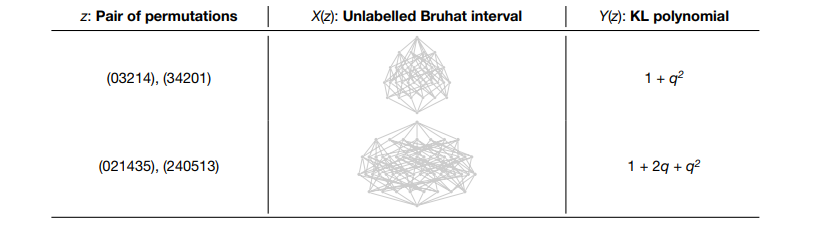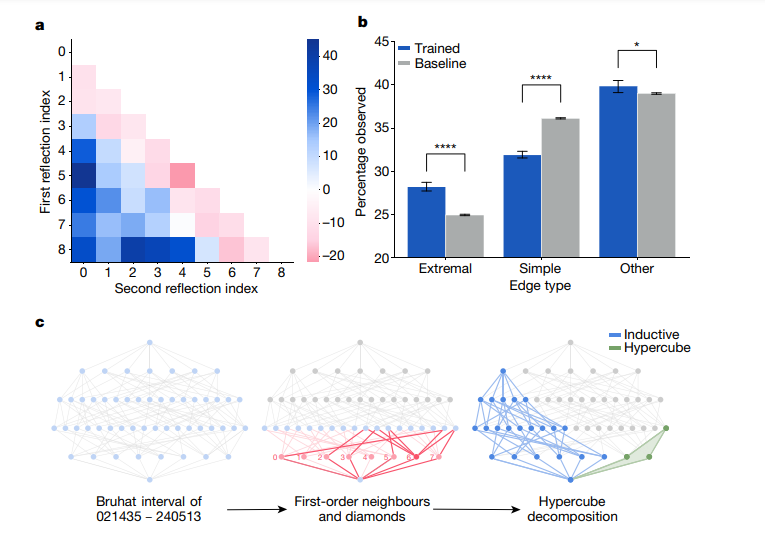### 6. 结论

1. Borwein, J. & Bailey, D. Mathematics by Experiment (CRC, 2008).
2. Birch, B. J. & Swinnerton-Dyer, H. P. F. Notes on elliptic curves. II. J. Reine Angew. Math. 1965, 79–108 (1965).
3. Carlson, J. et al. The Millennium Prize Problems (American Mathematical Soc., 2006).
4. Brenti, F. Kazhdan-Lusztig polynomials: history, problems, and combinatorial invariance. Sémin. Lothar. Combin. 49, B49b (2002).
5. Hoche, R. Nicomachi Geraseni Pythagorei Introductions Arithmeticae Libri 2 (In aedibus BG Teubneri, 1866).
6. Khovanov, M. Patterns in knot cohomology, I. Exp. Math. 12, 365–374 (2003).
7. Appel, K. I. & Haken, W. Every Planar Map Is Four Colorable Vol. 98 (American Mathematical Soc., 1989).
8. Scholze, P. Half a year of the Liquid Tensor Experiment: amazing developments Xena https://xenaproject.wordpress.com/2021/06/05/half-a-year-of-    the-liquid-tensor- experiment-amazing-developments/ (2021).
9. Fajtlowicz, S. in Annals of Discrete Mathematics Vol. 38 113–118 (Elsevier, 1988).
10. Larson, C. E. in DIMACS Series in Discrete Mathematics and Theoretical Computer Science Vol. 69 (eds Fajtlowicz, S. et al.) 297–318 (AMS & DIMACS, 2005).
11. Raayoni, G. et al. Generating conjectures on fundamental constants with the Ramanujan machine. Nature 590, 67–73 (2021).
12. MacKay, D. J. C. Information Theory, Inference and Learning Algorithms (Cambridge Univ. Press, 2003).
13. Bishop, C. M. Pattern Recognition and Machine Learning (Springer, 2006).
14. LeCun, Y., Bengio, Y. & Hinton, G. Deep learning. Nature 521, 436–444 (2015).
15. Raghu, M. & Schmidt, E. A survey of deep learning for scientific discovery. Preprint at https://arxiv.org/abs/2003.11755 (2020).
16. Wagner, A. Z. Constructions in combinatorics via neural networks. Preprint at https://arxiv.org/abs/2104.14516 (2021).
17. Peifer, D., Stillman, M. & Halpern-Leistner, D. Learning selection strategies in Buchberger’s algorithm. Preprint at https://arxiv.org/abs/2005.01917 (2020).
18. Lample, G. & Charton, F. Deep learning for symbolic mathematics. Preprint at https://arxiv.org/abs/1912.01412 (2019).
19. He, Y.-H. Machine-learning mathematical structures. Preprint at https://arxiv.org/abs/2101.06317 (2021).
20. Carifio, J., Halverson, J., Krioukov, D. & Nelson, B. D. Machine learning in the string landscape. J. High Energy Phys. 2017, 157 (2017).
21. Heal, K., Kulkarni, A. & Sertöz, E. C. Deep learning Gauss-Manin connections. Preprint at https://arxiv.org/abs/2007.13786 (2020).
22. Hughes, M. C. A neural network approach to predicting and computing knot invariants. Preprint at https://arxiv.org/abs/1610.05744 (2016).
23. Levitt, J. S. F., Hajij, M. & Sazdanovic, R. Big data approaches to knot theory: understanding the structure of the Jones polynomial. Preprint at
https://arxiv.org/abs/1912.10086 (2019).
24. Jejjala, V., Kar, A. & Parrikar, O. Deep learning the hyperbolic volume of a knot. Phys. Lett. B 799, 135033 (2019).
25. Tao, T. There’s more to mathematics than rigour and proofs Blog https://terrytao.wordpress.com/career-advice/theres-more-to-mathematics-than-rigour-and-proofs/ (2016).
26. Kashaev, R. M. The hyperbolic volume of knots from the quantum dilogarithm. Lett. Math. Phys. 39, 269–275 (1997).
27. Davies, A., Lackenby, M., Juhasz, A. & Tomašev, N. The signature and cusp geometry of hyperbolic knots. Preprint at arxiv.org (in the press).
28. Curtis, C. W. & Reiner, I. Representation Theory of Finite Groups and Associative Algebras Vol. 356 (American Mathematical Soc., 1966).
29. Brenti, F., Caselli, F. & Marietti, M. Special matchings and Kazhdan–Lusztig polynomials. Adv. Math. 202, 555–601 (2006).
30. Verma, D.-N. Structure of certain induced representations of complex semisimple Lie algebras. Bull. Am. Math. Soc. 74 , 160–166 (1968).
31. Braden, T. & MacPherson, R. From moment graphs to intersection cohomology. Math. Ann. 321, 533–551 (2001).
32. Blundell, C., Buesing, L., Davies, A., Veličković, P. & Williamson, G. Towards combinatorial invariance for Kazhdan-Lusztig polynomials. Preprint at arxiv.org (in the press).
33. Silver, D. et al. Mastering the game of Go with deep neural networks and tree search. Nature 529, 484–489 (2016).
34. Kanigel, R. The Man Who Knew Infinity: a Life of the Genius Ramanujan (Simon and Schuster, 2016).
35. Poincaré, H. The Value of Science: Essential Writings of Henri Poincaré (Modern Library, 1907).
36. Hadamard, J. The Mathematician’s Mind (Princeton Univ. Press, 1997).
37. Bronstein, M. M., Bruna, J., Cohen, T. & Veličković, P. Geometric deep learning: grids, groups, graphs, geodesics, and gauges. Preprint at  https://arxiv.org/abs/2104.13478 (2021).
38. Efroymson, M. A. in Mathematical Methods for Digital Computers 191–203 (John Wiley, 1960).
39. Xu, K. et al. Show, attend and tell: neural image caption generation with visual attention. In Proc. International Conference on Machine Learning 2048–2057 (PMLR, 2015).
40. Sundararajan, M., Taly, A. & Yan, Q. Axiomatic attribution for deep networks. In Proc. International Conference on Machine Learning 3319–3328 (PMLR, 2017).
41. Bradbury, J. et al. JAX: composable transformations of Python+NumPy programs (2018); https://github.com/google/jax
42. Martín A. B. A. D. I. et al. TensorFlow: large-scale machine learning on heterogeneous systems (2015); https://doi.org/10.5281/zenodo.4724125.
43. Paszke, A. et al. in Advances in Neural Information Processing Systems 32 (eds Wallach, H. et al.) 8024–8035 (Curran Associates, 2019).
44. Thurston, W. P. Three dimensional manifolds, Kleinian groups and hyperbolic geometry. Bull. Am. Math. Soc 6, 357–381 (1982).
45. Culler, M., Dunfield, N. M., Goerner, M. & Weeks, J. R. SnapPy, a computer program for studying the geometry and topology of 3-manifolds (2020); http://snappy.computop.org.
46. Burton, B. A. The next 350 million knots. In Proc. 36th International Symposium on Computational Geometry (SoCG 2020) (Schloss Dagstuhl-Leibniz-Zentrum für Informatik, 2020).
47. Warrington, G. S. Equivalence classes for the μ-coefficient of Kazhdan–Lusztig polynomials in Sn. Exp. Math. 20, 457–466 (2011).
48. Gilmer, J., Schoenholz, S. S., Riley, P. F., Vinyals, O. & Dahl, G. E. Neural message passing for quantum chemistry. Preprint at https://arxiv.org/abs/1704.01212 (2017).
49. Veličković, P., Ying, R., Padovano, M., Hadsell, R. & Blundell, C. Neural execution of graph algorithms. Preprint at https://arxiv.org/abs/1910.10593 (2019).

（参考文献可上下滑动查看）